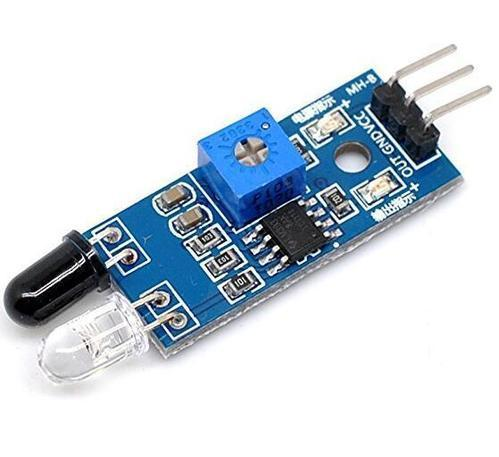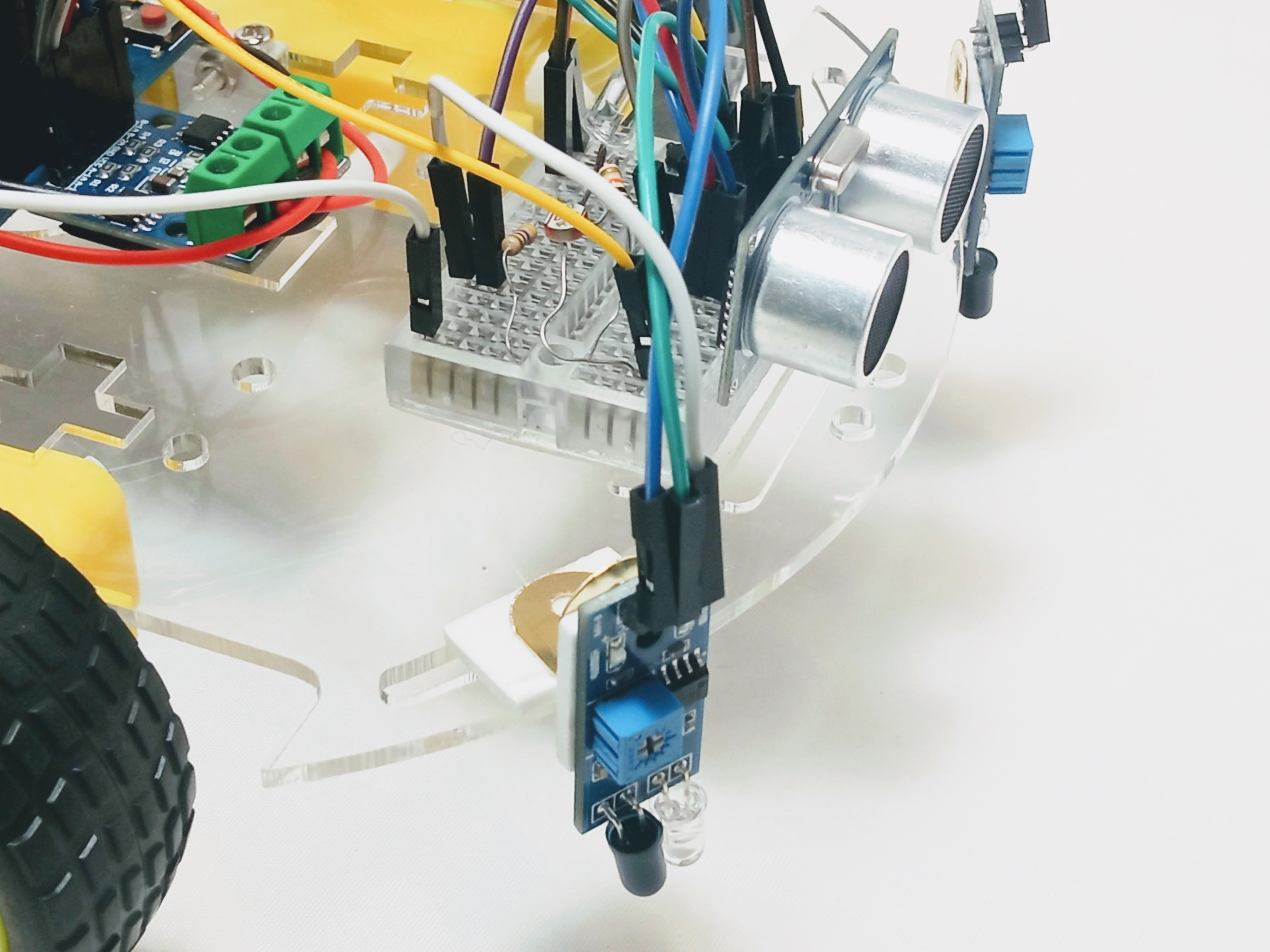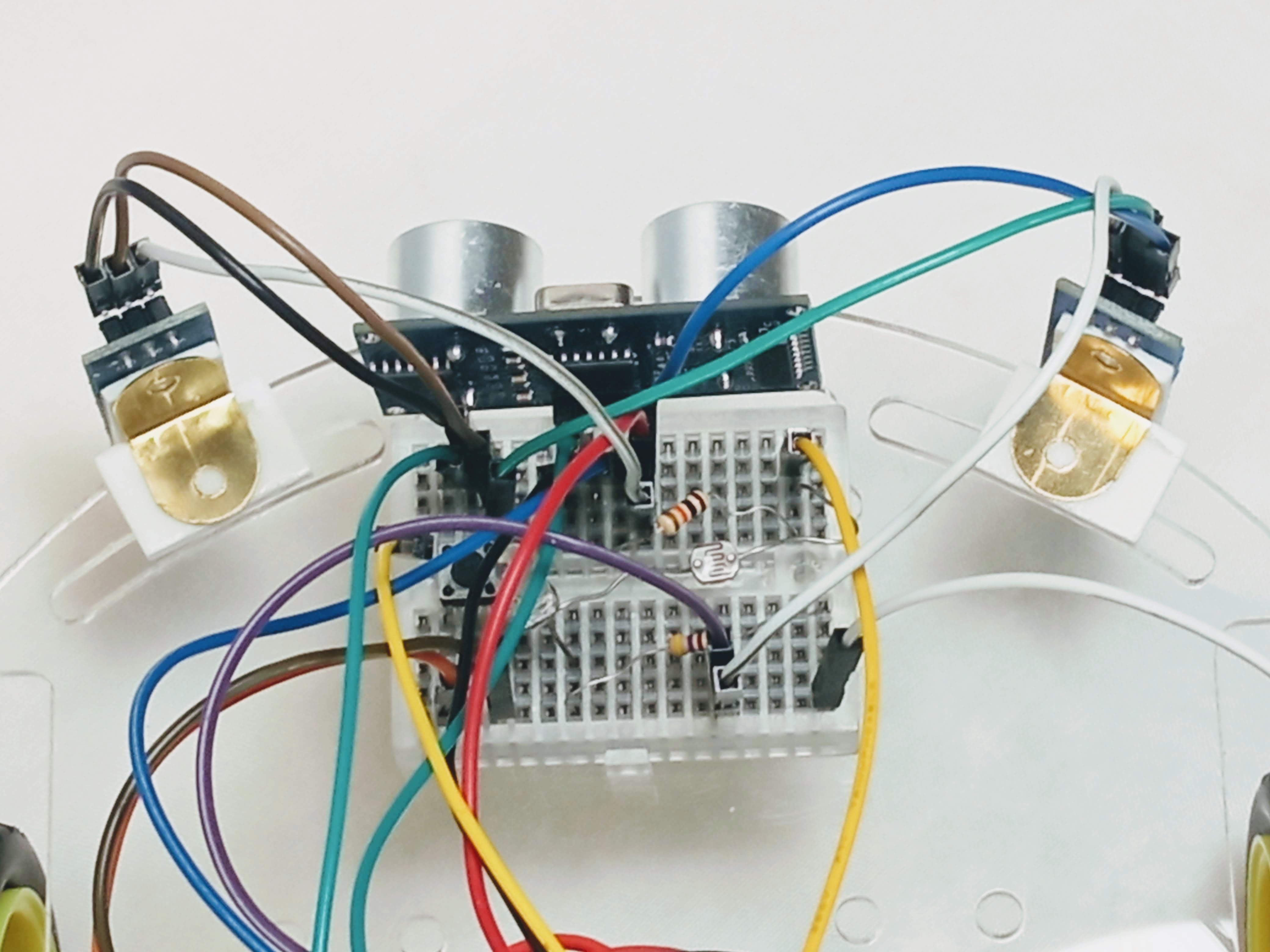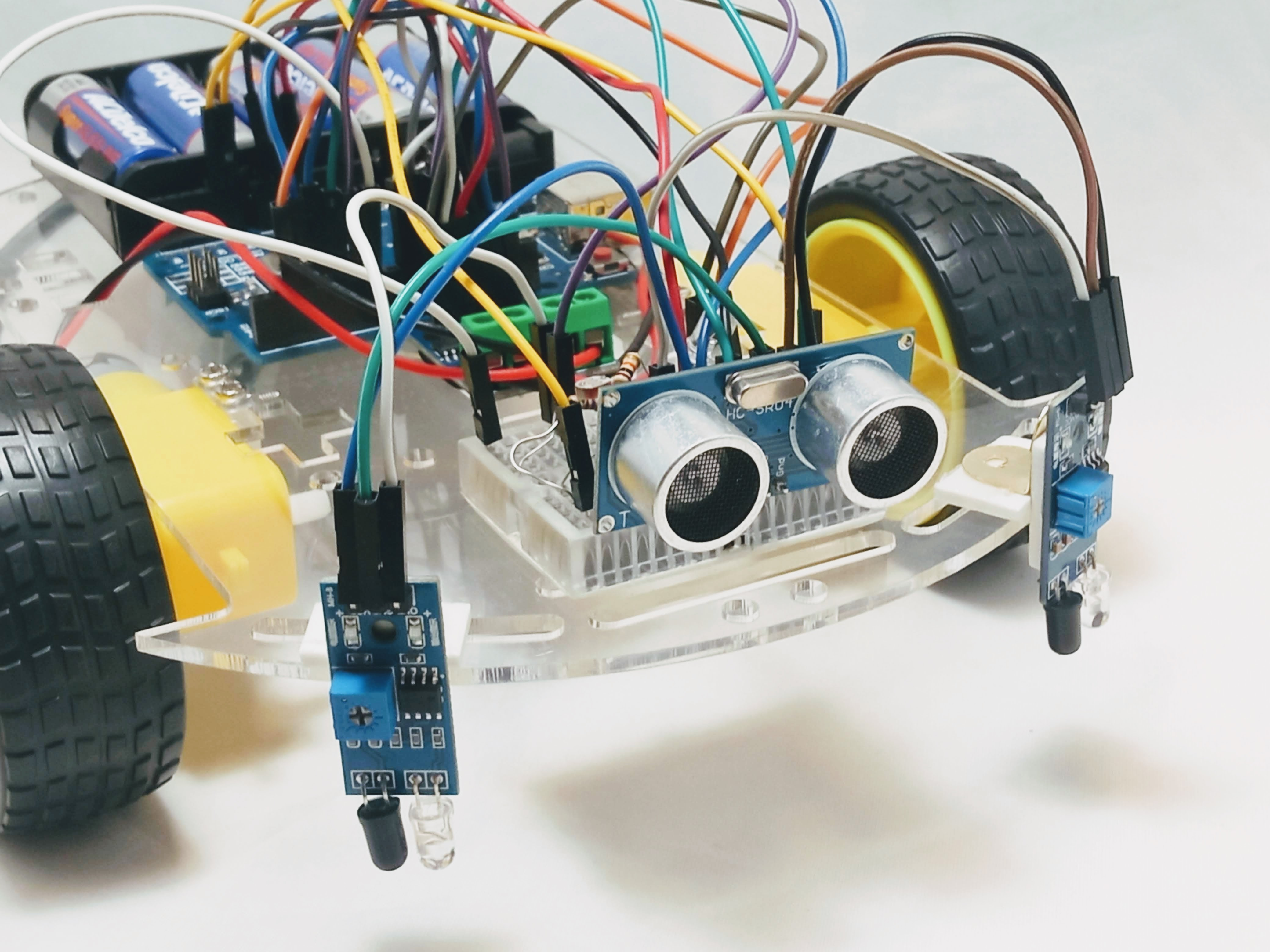# Lesson 15 · Line Following

Suggested Time: 60-75 minutes

## Lesson Plan

### Overview

In this section we’ll learn how to use IR sensors to get our robot to follow a line!

### What You’ll Need

Before we get started, let’s make sure that we have all the parts.

• 1 x Arduino Uno Compatible Board
• 2 x IR Sensor Modules
• 6 x Pin to Socket Arduino Wires
• 2 x Double-stick foam

### IR Sensor ModuleIR Sensor modules include two special LEDs. One LED is a IR transmitter (the clear one) and the other is a IR receiver (the dark one).

The transmitter sends a IR signal into the air. While the IR travels through the air, it will bounce off of near by objects, sending IR back to the module. The IR receiver looks for any IR signals that are bounced back and reports back to your robot if it does - which means that there is an object near by.

There is a caveat here, though. If IR hits black color, it won’t bounce back because the black color absorbs the IR. We can use this caveat to tell the different between white and black!

Use two modules, and you now have the ability to cause the robot to follow a line!

Mount your modules to the front bumper using double-stick foam.Note: Newer versions include 2 x 90 degree angle mounts to ease mounting. See photos below for mounting instructions.The IR modules have three pins: VCC, GND and OUT.

VCC and GND provide power to the module. OUT is the signal that goes to the controller board, which our code will look at to see whether we see white or black.

Let’s wire the IR modules to your Arduino-Compatible board using the following connections.

Left Sensor Arduino-Uno Compatible Board
VCC 5V
GND GND
OUT 5
Left Sensor Arduino-Uno Compatible Board
VCC 5V
GND GND
OUT 12
Right Sensor Arduino-Uno Compatible Board
VCC 5V
GND GND
OUT 6

### How to wire and code a single IR sensor

/*
Line following code
Video tutorial at: https://youtu.be/6JP4tyrF_q8
*/

int motb_pin1 = 3;
int motb_pin2 = 11;

int mota_pin1 = 9;
int mota_pin2 = 10;

int button_pin = 2;

int trig_pin = 4;
int echo_pin = 5;

int irsensor_pin = 6;

void setup() {

//-Control Motor B
pinMode(motb_pin1,OUTPUT);
pinMode(motb_pin2,OUTPUT);

//-Control Motor A
pinMode(mota_pin1,OUTPUT);
pinMode(mota_pin2,OUTPUT);

//- button
pinMode(button_pin,INPUT_PULLUP);

//-ultrasonic pin
pinMode(trig_pin,OUTPUT);
pinMode(echo_pin,INPUT);

//-IR sensor pin
pinMode(irsensor_pin,INPUT);

Serial.begin(9600);

}

//-sends sound out and receives sound
//-returns the distance in centimeters
int ultrasonic() {

long time;
float distance;

//-trigger a sound
// send out trigger signal
digitalWrite(trig_pin, LOW);
delayMicroseconds(2);
digitalWrite(trig_pin, HIGH);
delayMicroseconds(20);
digitalWrite(trig_pin, LOW);

//- a sound has gone out!!
//- wait for a sound to come back

time = pulseIn(echo_pin, HIGH);

//- calculate the distance in centimeters
distance = 0.01715 * time;

return distance;

}

//- turn 90 degrees
void turnRight() {

//- motor b is stopped
analogWrite(motb_pin1,0);
analogWrite(motb_pin2,0);

//- motor a moves forward
analogWrite(mota_pin1,255);
analogWrite(mota_pin2,0);

delay(600);

//- stop motor a
analogWrite(mota_pin1,0);
analogWrite(mota_pin2,0);

}

void tankTurn(int speeda, int speedb) {
//- motor a moves ... backwards
analogWrite(mota_pin1,0);
analogWrite(mota_pin2,speeda);

//- motor b moves forward
analogWrite(motb_pin1,0);
analogWrite(motb_pin2,speedb);

delay(1650);

//- stop both motors
stop();

}

void turnAround(int speeda, int speedb) {

//- motor a is stopped
analogWrite(mota_pin1,0);
analogWrite(mota_pin2,0);

//- motor b moves forward
analogWrite(motb_pin1,0);
analogWrite(motb_pin2,speedb);

delay(1650);

//- stop motor b
analogWrite(motb_pin1,0);
analogWrite(motb_pin2,0);

}

void turnLeft() {

//- motor a is stopped
analogWrite(mota_pin1,0);
analogWrite(mota_pin2,0);

//- motor b moves forward
analogWrite(motb_pin1,0);
analogWrite(motb_pin2,255);

delay(800);

//- stop motor b
analogWrite(motb_pin1,0);
analogWrite(motb_pin2,0);

}

void stop() {
//- motor a
analogWrite(mota_pin1,255);
analogWrite(mota_pin2,255);

//- motor b
analogWrite(motb_pin1,255);
analogWrite(motb_pin2,255);
}

void moveToWall(int speeda, int speedb) {

//- move forward!
//- motor a
analogWrite(mota_pin1,speeda);
analogWrite(mota_pin2,0);

//- motor b
analogWrite(motb_pin1,0);
analogWrite(motb_pin2,speedb);

//-stop when you hit a wall!!

int distance = ultrasonic();

while (distance > 5) {
//-do nothing except check distance
distance = ultrasonic();
}

//-stop!!!
stop();

}

void moveForward_no_distance(int speeda, int speedb) {
//- motor a
analogWrite(mota_pin1,speeda);
analogWrite(mota_pin2,0);

//- motor b
analogWrite(motb_pin1,0);
analogWrite(motb_pin2,speedb);
}

void forward() {
//- motor a
analogWrite(mota_pin1,150);
analogWrite(mota_pin2,0);

//- motor b
analogWrite(motb_pin1,0);
analogWrite(motb_pin2,150);
}

void tankRight() {
//- motor a
analogWrite(mota_pin1,150);
analogWrite(mota_pin2,0);

//- motor b
analogWrite(motb_pin1,150);
analogWrite(motb_pin2,0);
}

void tankLeft() {
//- motor a
analogWrite(mota_pin1,0);
analogWrite(mota_pin2,150);

//- motor b
analogWrite(motb_pin1,0);
analogWrite(motb_pin2,150);
}

void moveForward(int speeda, int speedb, int inches) {

int myDelay;

//- motor a
analogWrite(mota_pin1,speeda);
analogWrite(mota_pin2,0);

//- motor b
analogWrite(motb_pin1,0);
analogWrite(motb_pin2,speedb);

//- move forward the distance in inches

myDelay = inches*125;
delay(myDelay);

//- stop
stop();

}

void moveBackward(int speeda, int speedb) {

//- motor a
analogWrite(mota_pin1,0);
analogWrite(mota_pin2,speeda);

//- motor b
analogWrite(motb_pin1,speedb);
analogWrite(motb_pin2,0);

}

void loop() {

//- 1 -> senses black
//- 0 -> senses white
delay(100);

moveForward_no_distance(100,100);
}

}


### How to code line following using 2 sensors

Now that we have 1 sensor working, let’s go ahead and add the second sensor, and code our Rover to follow a line!/*
Line following code
Video tutorial at: https://youtu.be/6JP4tyrF_q8
*/

int motb_pin1 = 3;
int motb_pin2 = 11;

int mota_pin1 = 9;
int mota_pin2 = 10;

int button_pin = 2;

int trig_pin = 4;
int echo_pin = 5;

int right_sensor_pin = 6;
int left_sensor_pin = 12;

void setup() {

//-Control Motor B
pinMode(motb_pin1,OUTPUT);
pinMode(motb_pin2,OUTPUT);

//-Control Motor A
pinMode(mota_pin1,OUTPUT);
pinMode(mota_pin2,OUTPUT);

//- button
pinMode(button_pin,INPUT_PULLUP);

//-ultrasonic pin
pinMode(trig_pin,OUTPUT);
pinMode(echo_pin,INPUT);

//-IR sensor pin
pinMode(right_sensor_pin,INPUT);
pinMode(left_sensor_pin,INPUT);

Serial.begin(9600);

}

//-sends sound out and receives sound
//-returns the distance in centimeters
int ultrasonic() {

long time;
float distance;

//-trigger a sound
// send out trigger signal
digitalWrite(trig_pin, LOW);
delayMicroseconds(2);
digitalWrite(trig_pin, HIGH);
delayMicroseconds(20);
digitalWrite(trig_pin, LOW);

//- a sound has gone out!!
//- wait for a sound to come back

time = pulseIn(echo_pin, HIGH);

//- calculate the distance in centimeters
distance = 0.01715 * time;

return distance;

}

//- turn 90 degrees
void turnRight() {

//- motor b is stopped
analogWrite(motb_pin1,0);
analogWrite(motb_pin2,0);

//- motor a moves forward
analogWrite(mota_pin1,255);
analogWrite(mota_pin2,0);

delay(600);

//- stop motor a
analogWrite(mota_pin1,0);
analogWrite(mota_pin2,0);

}

void tankTurn(int speeda, int speedb) {
//- motor a moves ... backwards
analogWrite(mota_pin1,0);
analogWrite(mota_pin2,speeda);

//- motor b moves forward
analogWrite(motb_pin1,0);
analogWrite(motb_pin2,speedb);

delay(1650);

//- stop both motors
stop();

}

void turnAround(int speeda, int speedb) {

//- motor a is stopped
analogWrite(mota_pin1,0);
analogWrite(mota_pin2,0);

//- motor b moves forward
analogWrite(motb_pin1,0);
analogWrite(motb_pin2,speedb);

delay(1650);

//- stop motor b
analogWrite(motb_pin1,0);
analogWrite(motb_pin2,0);

}

void turnLeft() {

//- motor a is stopped
analogWrite(mota_pin1,0);
analogWrite(mota_pin2,0);

//- motor b moves forward
analogWrite(motb_pin1,0);
analogWrite(motb_pin2,255);

delay(800);

//- stop motor b
analogWrite(motb_pin1,0);
analogWrite(motb_pin2,0);

}

void stop() {
//- motor a
analogWrite(mota_pin1,255);
analogWrite(mota_pin2,255);

//- motor b
analogWrite(motb_pin1,255);
analogWrite(motb_pin2,255);
}

void moveToWall(int speeda, int speedb) {

//- move forward!
//- motor a
analogWrite(mota_pin1,speeda);
analogWrite(mota_pin2,0);

//- motor b
analogWrite(motb_pin1,0);
analogWrite(motb_pin2,speedb);

//-stop when you hit a wall!!

int distance = ultrasonic();

while (distance > 5) {
//-do nothing except check distance
distance = ultrasonic();
}

//-stop!!!
stop();

}

void moveForward_no_distance(int speeda, int speedb) {
//- motor a
analogWrite(mota_pin1,speeda);
analogWrite(mota_pin2,0);

//- motor b
analogWrite(motb_pin1,0);
analogWrite(motb_pin2,speedb);
}

void forward() {
//- motor a
analogWrite(mota_pin1,150);
analogWrite(mota_pin2,0);

//- motor b
analogWrite(motb_pin1,0);
analogWrite(motb_pin2,150);
}

void tankRight() {
//- motor a
analogWrite(mota_pin1,150);
analogWrite(mota_pin2,0);

//- motor b
analogWrite(motb_pin1,150);
analogWrite(motb_pin2,0);
}

void tankLeft() {
//- motor a
analogWrite(mota_pin1,0);
analogWrite(mota_pin2,150);

//- motor b
analogWrite(motb_pin1,0);
analogWrite(motb_pin2,150);
}

void moveForward(int speeda, int speedb, int inches) {

int myDelay;

//- motor a
analogWrite(mota_pin1,speeda);
analogWrite(mota_pin2,0);

//- motor b
analogWrite(motb_pin1,0);
analogWrite(motb_pin2,speedb);

//- move forward the distance in inches

myDelay = inches*125;
delay(myDelay);

//- stop
stop();

}

void moveBackward(int speeda, int speedb) {

//- motor a
analogWrite(mota_pin1,0);
analogWrite(mota_pin2,speeda);

//- motor b
analogWrite(motb_pin1,speedb);
analogWrite(motb_pin2,0);

}

void loop() {

//- 1 -> senses black
//- 0 -> senses white

if (leftStatus == 1 && rightStatus == 0) {
//-left sensor sees black
//-tank left
tankLeft();
}

if (rightStatus == 1 && leftStatus == 0) {
//- right sensor sees black
//- tank right
tankRight();
}

if (leftStatus == 0 && rightStatus == 0) {
//- both sensors see white
//- go straight
forward();
}

if (leftStatus == 1 && rightStatus == 1) {
//- both sensors see black/nothing
//- stop
stop();
}

}


### Troubleshooting Tips

• Your rover may be heading in the wrong direction when it sees the line. If that’s the case, you probably have your left/right sensor mixed up. Check your wiring and code.
• You may find that your rover reacts too slowly and and veers off the track before it can turn. If that’s the case, try slowing down your racer by moving at half speed. See lesson 8 if you need a refresher on how to do that.